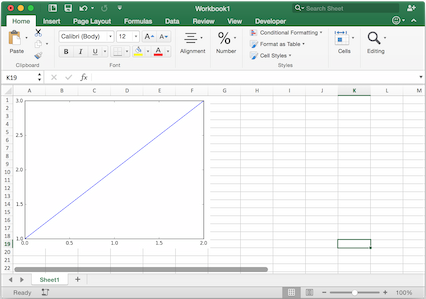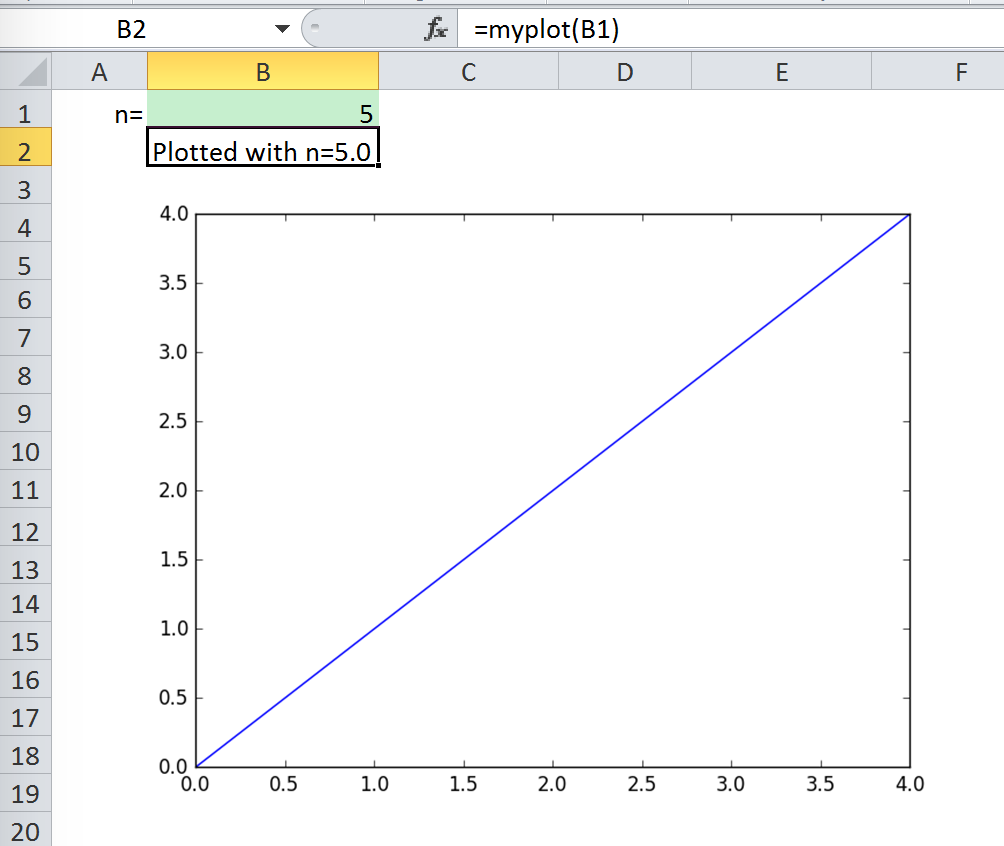# Matplotlib & Plotly Charts¶

## Matplotlib¶

Using `pictures.add()`, it is easy to paste a Matplotlib plot as picture in Excel.

### Getting started¶

The easiest sample boils down to:

```import matplotlib.pyplot as plt
import xlwings as xw

fig = plt.figure()
plt.plot([1, 2, 3])

sheet = xw.Book().sheets
```Note

If you set `update=True`, you can resize and position the plot on Excel: subsequent calls to `pictures.add()` with the same name (`'MyPlot'`) will update the picture without changing its position or size.

### Full integration with Excel¶

Calling the above code with RunPython and binding it e.g. to a button is straightforward and works cross-platform.

However, on Windows you can make things feel even more integrated by setting up a UDF along the following lines:

```@xw.func
def myplot(n, caller):
fig = plt.figure()
plt.plot(range(int(n)))
return 'Plotted with n={}'.format(n)
```

If you import this function and call it from cell B2, then the plot gets automatically updated when cell B1 changes:### Properties¶

Size, position and other properties can either be set as arguments within `pictures.add()`, or by manipulating the picture object that is returned, see `xlwings.Picture()`.

For example:

```>>> sht = xw.Book().sheets
left=sht.range('B5').left, top=sht.range('B5').top)
```

or:

```>>> plot = sht.pictures.add(fig, name='MyPlot', update=True)
>>> plot.height /= 2
>>> plot.width /= 2
```

### Getting a Matplotlib figure¶

Here are a few examples of how you get a matplotlib `figure` object:

• via PyPlot interface:

```import matplotlib.pyplot as plt
fig = plt.figure()
plt.plot([1, 2, 3, 4, 5])
```

or:

```import matplotlib.pyplot as plt
plt.plot([1, 2, 3, 4, 5])
fig = plt.gcf()
```
• via object oriented interface:

```from matplotlib.figure import Figure
fig = Figure(figsize=(8, 6))
ax.plot([1, 2, 3, 4, 5])
```
• via Pandas:

```import pandas as pd
import numpy as np

df = pd.DataFrame(np.random.rand(10, 4), columns=['a', 'b', 'c', 'd'])
ax = df.plot(kind='bar')
fig = ax.get_figure()
```

## Plotly static charts¶

### Prerequisites¶

In addition to `plotly`, you will need `kaleido`, `psutil`, and `requests`. The easiest way to get it is via pip:

```\$ pip install kaleido psutil requests
```

or conda:

```\$ conda install -c conda-forge python-kaleido psutil requests
```

### How to use¶

It works the same as with Matplotlib, however, rendering a Plotly chart takes slightly longer. Here is a sample:

```import xlwings as xw
import plotly.express as px

# Plotly chart
df = px.data.iris()
fig = px.scatter(df, x="sepal_width", y="sepal_length", color="species")